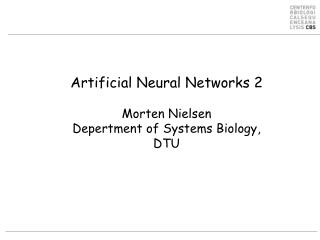DownloadDownload PresentationArtificial Neural Networks 2 Morten Nielsen Depertment of Systems Biology , DTU

# Artificial Neural Networks 2 Morten Nielsen Depertment of Systems Biology , DTU

Download Presentation## Artificial Neural Networks 2 Morten Nielsen Depertment of Systems Biology , DTU

- - - - - - - - - - - - - - - - - - - - - - - - - - - E N D - - - - - - - - - - - - - - - - - - - - - - - - - - -
##### Presentation Transcript

1. Artificial Neural Networks 2Morten NielsenDepertment of Systems Biology,DTU

2. Outline • Optimization procedures • Gradient decent (this you already know) • Network training • back propagation • cross-validation • Over-fitting • examples

3. Neural network. Error estimate Linear function I1 I2 w1 w2 o

4. Neural networks

5. Gradient decent (from wekipedia) Gradient descent is based on the observation that if the real-valued function F(x) is defined and differentiable in a neighborhood of a point a, then F(x) decreases fastest if one goes from a in the direction of the negative gradient of F at a. It follows that, if for  > 0 a small enough number, then F(b)<F(a)

8. Gradient decent. Example Weights are changed in the opposite direction of the gradient of the error Linear function I1 I2 w1 w2 o

9. What about the hidden layer?

10. Hidden to output layer

11. Hidden to output layer

12. Hidden to output layer

13. Input to hidden layer

14. Input to hidden layer

15. Input to hidden layer

16. Summary

17. Or

18. Or Ii=X[k] Hj=X[j] Oi=X[i]

19. Can you do it your self? I1=1 I2=1 v21=-1 v12=1 v11=1 v22=1 h1 H1 h2 H2 w2=1 w1=-1 o O What is the output (O) from the network? What are the wij and vjk values if the target value is 0 and =0.5?

20. Can you do it your self (=0.5). Has the error decreased? After Before I1=1 I2=1 I1=1 I2=1 v21=-1 v12=1 v21= v12= v11=1 v22=1 V11= v22=. h2= H2= h1= H1= h1= H1= h2= H2= w2=1 w1=-1 w2= w1= o= O= o= O=

21. Sequence encoding • Change in weight is linearly dependent on input value • “True” sparse encoding is therefore highly inefficient • Sparse is most often encoded as • +1/-1 or 0.9/0.05

22. Training and error reduction Size matters 

23. Neural network training • A Network contains a very large set of parameters • A network with 5 hidden neurons predicting binding for 9meric peptides has more than 9x20x5=900 weights • Over fitting is a problem • Stop training when test performance is optimal Temperature years

24.  What is going on? Temperature years

25. Examples Train on 500 A0201 and 60 A0101 binding data Evaluate on 1266 A0201 peptides NH=1: PCC = 0.77 NH=5: PCC = 0.72

26. Neural network training. Cross validation Cross validation Train on 4/5 of data Test on 1/5 => Produce 5 different neural networks each with a different prediction focus

27. Neural network training curve Maximum test set performance Most cable of generalizing

28. 5 fold training Which network to choose?

29. 5 fold training

30. How many folds? • Cross validation is always good!, but how many folds? • Few folds -> small training data sets • Many folds -> small test data sets • Example from Tuesdays exercise • 560 peptides for training • 50 fold (10 peptides per test set, few data to stop training) • 2 fold (280 peptides per test set, few data to train) • 5 fold (110 peptide per test set, 450 per training set)

31. Problems with 5fold cross validation • Use test set to stop training, and test set performance to evaluate training • Over-fitting? • If test set is small yes • If test set is large no • Confirm using “true” 5 fold cross validation • 1/5 for evaluation • 4/5 for 4 fold cross-validation

32. Conventional 5 fold cross validation

33. “True” 5 fold cross validation

34. When to be careful • When data is scarce, the performance obtained used “conventional” versus “true” cross validation can be very large • When data is abundant the difference is small, and “true” cross validation might even be higher than “conventional” cross validation due to the ensemble aspect of the “true” cross validation approach

35. NetMHCpan Do hidden neurons matter? • The environment matters

36. Context matters • FMIDWILDA YFAMYGEKVAHTHVDTLYVRYHYYTWAVLAYTWY 0.89 A0201 • FMIDWILDA YFAMYQENMAHTDANTLYIIYRDYTWVARVYRGY 0.08 A0101 • DSDGSFFLY YFAMYGEKVAHTHVDTLYVRYHYYTWAVLAYTWY 0.08 A0201 • DSDGSFFLY YFAMYQENMAHTDANTLYIIYRDYTWVARVYRGY 0.85 A0101

37. Example

38. Summary • Gradient decent is used to determine the updates for the synapses in the neural network • Some relatively simple math defines the gradients • Networks without hidden layers can be solved on the back of an envelope (SMM exercise) • Hidden layers are a bit more complex, but still ok • Always train networks using a test set to stop training • Be careful when reporting predictive performance • Use “true” cross-validation for small data sets • And hidden neurons do matter (sometimes)

39. And some more stuff for the long cold winter nights • Can it might be made differently?

40. Predicting accuracy • Can it be made differently? Reliability

41. Making sense of ANN weights • Identification of position specific receptor ligand interactions by use of artificial neural network decomposition. An investigation of interactions in the MHC:peptide system Master these by FrederikOtzen Bagger

42. Making sense of ANN weights

43. Making sense of ANN weights

44. Making sense of ANN weights

45. Making sense of ANN weights

46. Making sense of ANN weights

47. Making sense of ANN weights